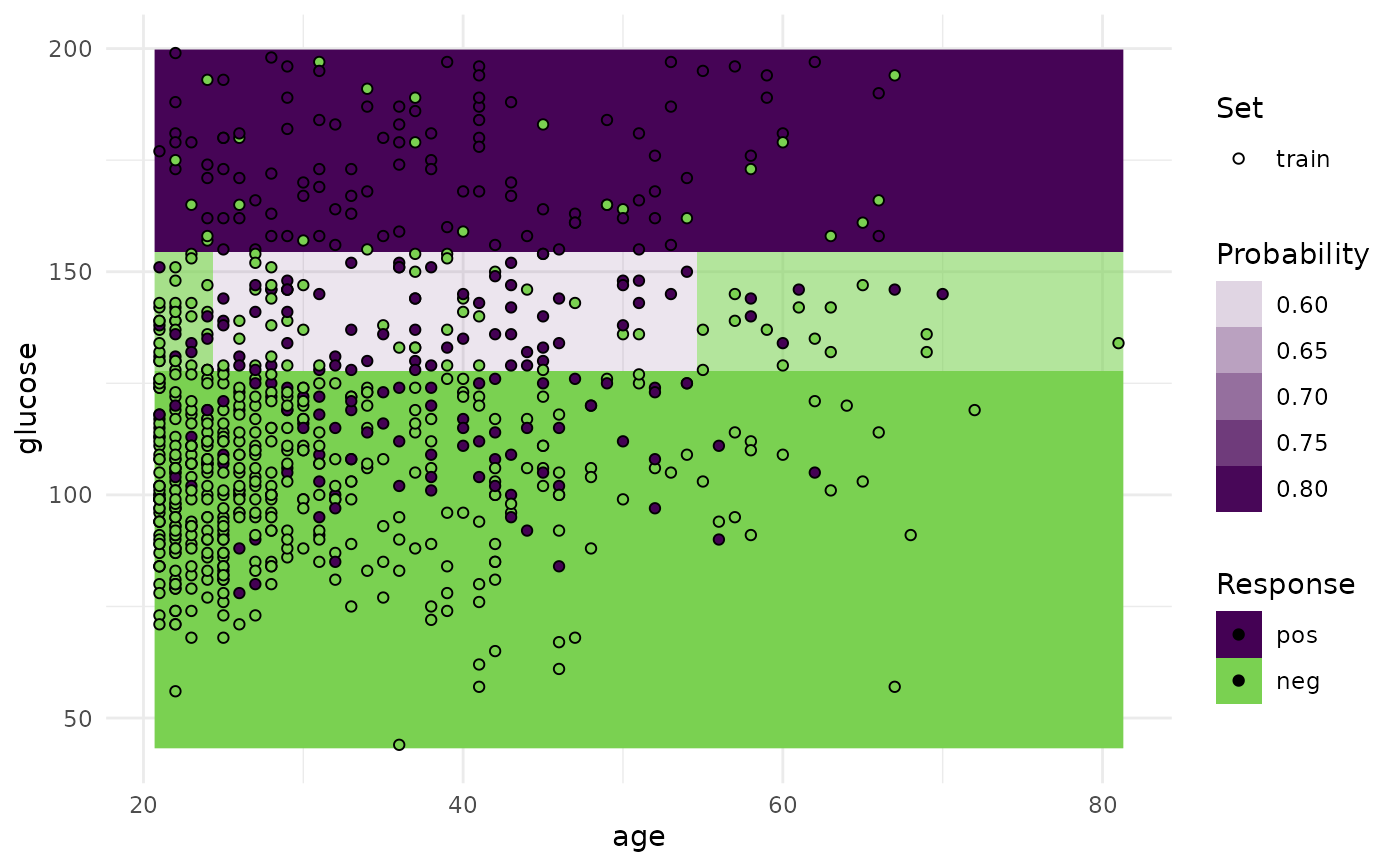Visualizations for the mlr3::Prediction of a single mlr3::Learner on a single mlr3::Task.

• For classification we support tasks with exactly two features and learners with predict_type set to "response" or "prob".

• For regression we support tasks with one or two features. For tasks with one feature we print confidence bounds if the predict type of the learner was set to "se". For tasks with two features the predict type will be ignored.

Note that this function is a wrapper around autoplot.ResampleResult() for a temporary mlr3::ResampleResult using mlr3::mlr_resamplings_holdout with ratio 1 (all observations in the training set).

## Usage

plot_learner_prediction(learner, task, grid_points = 100L, expand_range = 0)

## Arguments

learner

grid_points

(integer(1))
Resolution of the grid. For factors, ordered and logicals this value is ignored.

expand_range

(numeric(1))
Expand the prediction range for numerical features.

## Value

ggplot2::ggplot().

## Examples

if (requireNamespace("mlr3")) {
library(mlr3)
library(mlr3viz)

#> Warning: Removed 5 rows containing missing values (geom_point()).